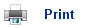# Calculating Percentage Yield

• It is very rare for an organic reaction to give 100% of the required product.
• The amount of product obtained is hardly ever as much as predicted by the reaction.
• This is related to the fact that most organic reactions are accompanied by side-reactions that lead to the formation of minor products.
• For example, a sample of lactic acid can be synthesised in the laboratory from ethanal in a series of steps: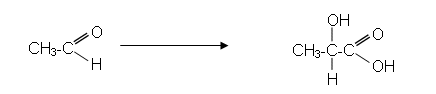• Suppose a chemist started with 5.00g of ethanal, if all of it reacted to form lactic acid as shown in the synthesis, then: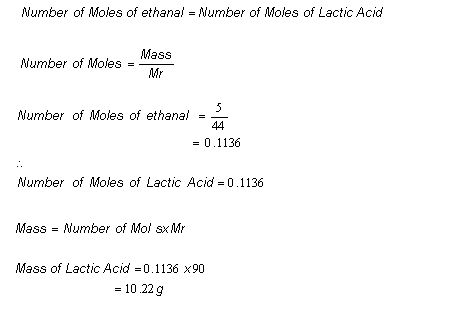• However, when the chemist carried out the reaction, only 7.02g of lactic acid was obtained.
• Therefore the percentage yield of lactic acid equalled: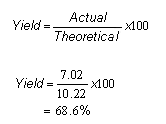• Usually a reaction is given a maximum percentage yield; as the name suggests, this is the highest percentage of theoretical product that can practically be obtained.
• A reaction yield of 90% of the theoretical possible would be considered excellent. 80% would be very good. Even a yield of 50% is considered adequate.
• Suppose a synthesis involved three steps, each of which gave a 90% yield of product: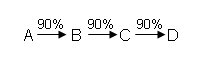• The overall percentage yield for the synthesis would be equal to: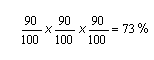• However, if each step only provided a 50% yield, then the overall yield would be only 12.5%.

## Useful books for revision

Revise A2 Chemistry for Salters (OCR A Level Chemistry B)Salters (OCR) Revise A2 ChemistryHome